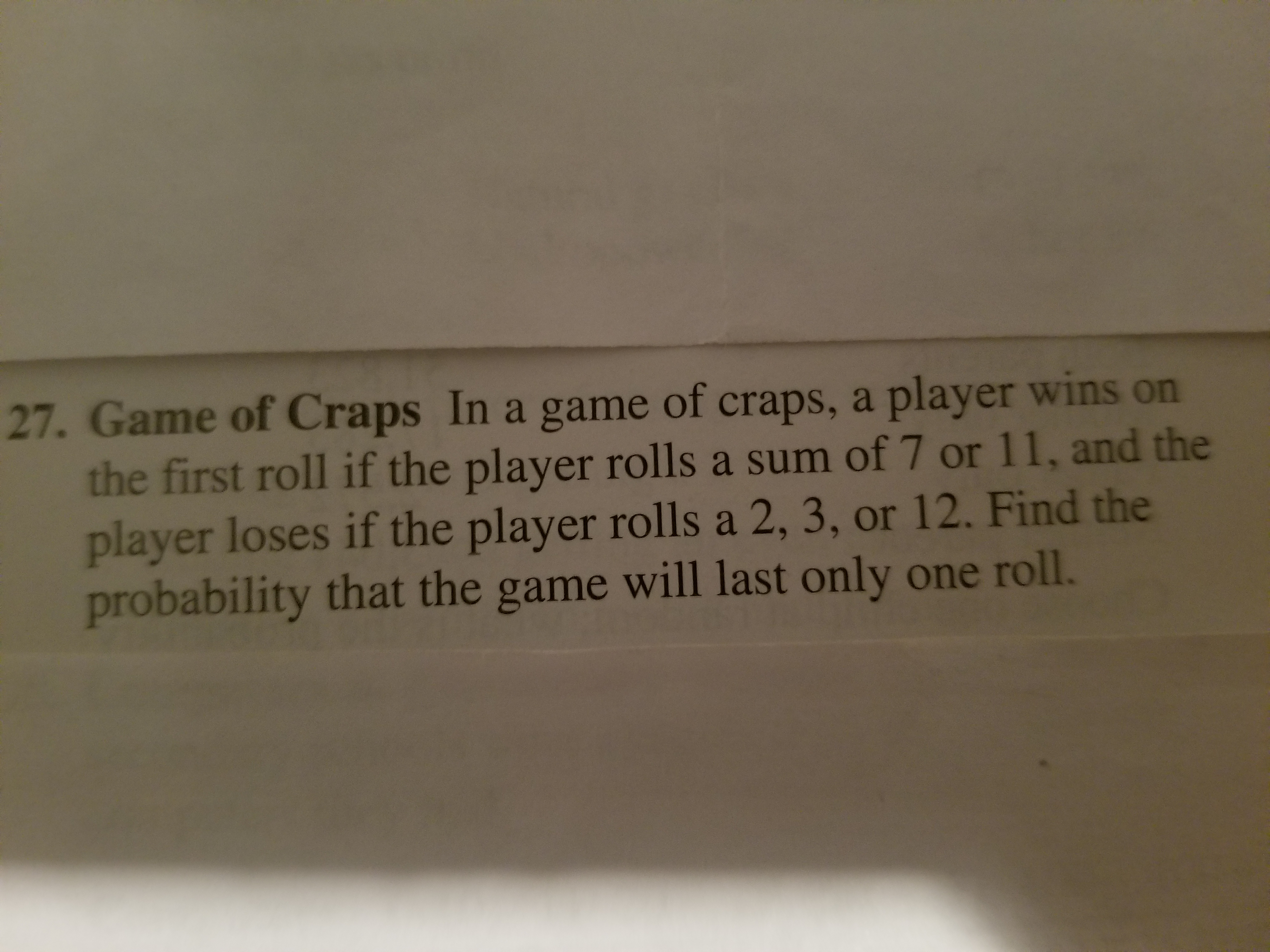# 27. Game of Craps In a game of craps, a player wins onthe first roll if the player rolls a sum of 7 or 11, and theplayer loses if the player rolls a 2, 3, or 12. Find theprobability that the game will last only one roll.

Question
140 viewshelp_outlineImage Transcriptionclose27. Game of Craps In a game of craps, a player wins on the first roll if the player rolls a sum of 7 or 11, and the player loses if the player rolls a 2, 3, or 12. Find the probability that the game will last only one roll. fullscreen
check_circle

Step 1

Given a player wins if he rolls a sum of 7 or 11 when rolled a pair of dice.

A player loses if he rolls a sum of 2, 3 or 12.

We need t find the probability that game will last only one roll. So here we need to find the probability of either win or loss in first roll, such that game will last only one roll.

If two events A,B are mutually exclusive then we can say that both events does not occur simultaneously. So P(A or B) = P(A) + P(B). Here win and loss are both mutually exclusive events. So we can say

P(Win or loss) = P(win)+P(loss)

Step 2

P(Win) = probability of rolling 7 or 11.

When a die is rolled number of possibilities = 6

When two dice are rolled, number of possibilities = 6*6 = 36.

The total number of ways of rolling a 7 when we roll two dice = 6 ways(as shown below)

The total number of ways of rolling a 11 when we roll two dice = 2 ways...

### Want to see the full answer?

See Solution

#### Want to see this answer and more?

Solutions are written by subject experts who are available 24/7. Questions are typically answered within 1 hour.*

See Solution
*Response times may vary by subject and question.
Tagged in

### Measures